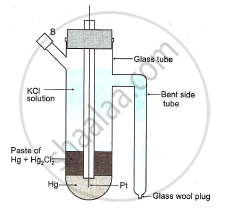# Derive a Relation Between δH and δU for a Chemical Reaction. Draw Neat Labelled Diagram of Calomel Electrode. - Chemistry

Sum

Derive a relation between ΔH and ΔU for a chemical reaction. Draw neat labelled diagram of calomel electrode. Resistance and conductivity of a cell containing 0.001 M KCI solution at 298K are 1500Ω and 1.46x10-4 S.cm-1 respectively.

#### Solution

Relation between ΔH and ΔU

The heat of reaction is given by enthalpy change

ΔH=H2 - H1

by defination H= U+PV

H1=U1+P1V1

H2=U2+P2V2

ΔH = (U1+P1V1) - (U2+P2V2)

=(U2-U1)+(P2V2-P1V1)

=ΔU+(P2V2-P1V1)(∵ΔU=U2-U1)

since PV=nRT

for initial state P1V1=n1RT

for final state P2V2=n2RT

P2V2-P1V1=n2RT-n1RT

=(n2-n)RT

=ΔnRT

where Δn = number of moles of gaseous product - number of moles of gaseous reactant.

 ΔH=ΔU+ΔnRT

Diagram : Standard calomel electrodeData: R 1500Ω

C=0.001M

k= 1.46x10-4Scm-1

To find: b = ?

Solution : k=b/R

b=kxR

=1.46x10-4x1500

b=2190x10-4 cm-1

 b = 0.219cm-1
Concept: Electrochemical Cells
Is there an error in this question or solution?
2013-2014 (March)

Share